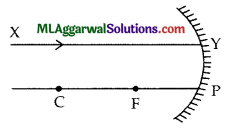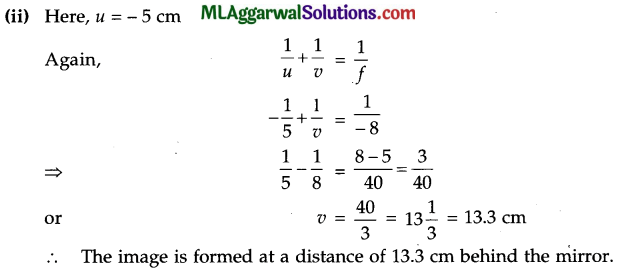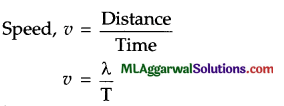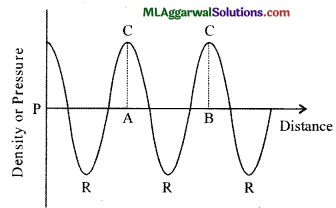## ICSE Class 9 Physics Sample Question Paper 6 with Answers

Section -1
(Attempt all questions from this section)

Question 1.
(a) A screw gauge has pitch of 1.0 mm and 200 divisions on the circular scale. Will it be possible to increase the accuracy of the gauge arbitrarily by increasing the number of divisions on the circular scale ?

(b) Fill in the blanks :
(i) Zero error is positive when the zero of vernier scale lies to the of the zero of the main scale, when the two jaws of the vernier are in contact.
(ii) Zero error is negative when the zero of vernier scale lies to the of the
zero of the main scale, when the two jaws of the vernier are in contact.

(c) Can a body have constant speed and still be accelerating ?(d) Two similar vehicles are moving with same velocity on the road, such that one of them is loaded and the other is empty. Which of the two vehicles will require larger force to stop ?

(e) If the earth attracts an apple, does the apple also attract the earth ? If so, why does the earth not move towards the apple ?
Number of divisions on the circular scale $$\text { Least Count }=\frac{\text { Pitch }}{\text { Number of divisions on the circular scale }}$$
Hence, on increasing the number of divisions on the circular scale, the least count of the gauge decreases, i.e., accuracy of gauge can be increased by increasing the number of divisions on circular scale.

(b) (i) Right
(ii) Left

(c) Yes, a body can have constant speed and still be accelerating. When the body moves along a circular path with uniform speed, it possesses centripetal acceleration.

(d) A larger force is required to stop the loaded vehicle than the empty one. This is because the loaded vehicle has a greater momemtum than the empty vehicle as the mass of the loaded vehicle is more than that of the empty vehicle. Thus, it requires a greater force to stop.

(e) Yes, the apple attracts the earth with the same force with which the earth attracts the apple. Due to the large mass of the earth, the acceleration produced in it is negligible, and goes unnoticed. Hence, the earth does not seem to move towards the apple.Question 2.
(a) Why do the bodies of deep sea fishes burst on bringing them above the sea level ?
(b) Why does a ship sink to a greater depth in river water than in sea water ?
(c) State two uses of biogas.
(d) Name the S.I. unit of heat and how is it related to the unit calorie ?
(e) A jar has water with 25 cm of height. Find the pressure of water at the bottom of the jar, ignore the atmospheric pressure and use the acceleration due to gravity g = 10 ms-2, and the density of water 1000 kg m-3.
(a) The pressure in the lower levels of sea is a lot greater than the atmospheric pressure. To thrive in such high pressure, the blood of deep sea fishes contains dissolved oxygen at a very high pressure as compared to atmospheric pressure. Thus, when the fishes are brought to sea level, the difference in pressure of oxygen within their bodies and atmosphere bursts open their bodies.

(b) The density of sea water, because of the presence of impurities like salt etc., is greater than that of river water. Therefore, a lesser volume of the ship will be immersed in sea water to balance its weight and hence, the ships sinks to greater depths in river water.(c) Two uses of biogas are as follows :
(i) Biogas is used for cooking food.
(ii) Biogas is being used for street-lighting.
(iii) The S.I. unit of heat is joule (J).

(d) 1 J = 0.24 cal (nearly).

(e) Given : h = 25 cm = 0.25 m, g = 10 ms-2, ρ = 1000 kg m-3
Using P = hρg
P = 0.25 x 1000 x 10
Pa = 2500 Pa

Question 3.
(a) A ray of light XY is incident on a mirror as shown in the figure. The angle of incidence for this ray is the angle between it and the line joining one of the two other points in the figure. Name these two points and show the course of rays after reflection.(b) You have a spherical mirror. The image of an object placed in front of the mirror is virtual. If the position of the object is changed, the image remains virtual and erect. Is the spherical mirror concave or convex ? Give reason.
(c) For driving a car what type of mirror would you prefer to see the traffic at your back and why ?
(d) Complete the following sentence :
(e) A plane mirror produces a………………………… and an…………………. image.
(f) In case of a convex mirror, if the object is moved away from the surface of the mirror, how does the position and size of the image change ?
(a) The angle of incidence is the angle between the incident ray and the normal at the point of incidence. A line joining the centre of curvature and the point of incidence is a normal at the point of incidence. Thus, the given two points are C and Y and the course of the rays is shown in the given figure.
C and F are respectively centre of curvature and focus.(b) A convex mirror always produces a virtual and erect image irrespective of the position of the object in front of the mirror. So the given spherical mirror is convex in nature.(c) We prefer a convex mirror for observing the traffic behind us because its field of view is much larger than the plane mirror.

(d) Virtual; erect

(e) As the object is moved away from a convex mirror, the distance of the virtual image formed behind it, from the mirror, increases (between pole and focus), i.e., the image shifts from the pole towards the focus and the size of the image gradually decreases. When the object is at infinity, the image is at its focus.

Question 4.
(a) You are given a primary and a secondary cell of the same e.m.f. From which cell, will you be able to draw a larger current and why ?
(b) Fill in the blanks :
(i) A source of direct current or e.m.f. is an arrangement which can bring about the of free electrons in a conductor in a particular direction.
(ii) An eletrochemical cell or cell is an arrangement which converts………………………. into electrical energy.
(c) Give four examples of primary cells.
(d) What are natural magnets ? Give example.
(e) Define magnetic equator.
(a) The internal resistance of a secondary cell is less than that of a primary cell and more current flows when resistance is less. So, the secondary cell will provide a greater amount of current.
(b) (i) Motion,
(ii) Chemical energy
(c) Examples of primary cells are – (1) Simple voltaic cell, (2) Daniel cell, (3) Leclanche cell, (4) Dry cell.
(d) The natural magnets are the ones which occur naturally in nature. For example, lodestone.
(e) The line joining the points on the earth where a magnetic needle points horizontally is called the magnetic equator.

Section- II
(Attempt any four questions from this section)

Question 5.
(a) State the difference between speed and velocity.
(b) Mention three uses of velocity time graphs.
(c) How is the time period of a simple pendulum affected in the following situations :
(i) Amplitude of the pendulum is increased.
(ii) The length of the pendulum is doubled.
(iii) The brass bob is replaced with an identical aluminium bob.
(iv) The value of g is made one-fourth.
(a) Speed is the rate of change of distance. It is a scalar quantity and is always positive. Velocity is the rate of change of displacement. It is a vector quantity and can be positive, negative or zero.(b) Velocity-time graphs have the following uses :
(1) To determine the total distance travelled by a particle.
(2) To determine instantaneous velocity of the particle.
(3) To determine the acceleration of the object.

(c) The time period of a pendulum is given by the expression $$\mathrm{T}=2 \pi \sqrt{\frac{\mathrm{L}}{g}}$$
(i) The time period of a pendulum does not depend upon its amplitude, hence there is no change.
(ii) The time period of a pendulum is directly proportional to the square root of its length. When length is increased, the time period will also increase. Hence, when the effective length is doubled, time period increases times.
(iii) The time period of a pendulum does not depend upon its amplitude, hence there is no change.
(iv) The time period of a pendulum is directly proportional to the square root of its length. When length is increased, the time period will also increase. Hence, when the effective length is doubled, time period increases times.

Question 6.
(a) State three factors that prevents us in making use of solar cell panels to meet all our domestic needs.
(b) State three steps that can help in reducing global warming.
(c) State the weather forecast in the following situations :
(i) Air is humid and barometer pressure falls suddenly.
(ii) Barometric pressure rises steeply.
(iii) Barometric pressure does not change.
(iv) Barometric pressure falls gradually over a number of days.
(a) The following factors prevent the use of solar cell panels to meet all our domestic needs of electricity :
(1) High cost of installation.
(2) Limited availability of special grade silicon to make solar cells.
(3) The effective energy obtained is not always constant and changes will seasons.

(b) Steps to reduce global warming are :
(i) By replacing a regular incandescent light bulb with a compact fluorescent light bulb (CFL) or LED.
(ii) By choosing energy efficient appliances when making new purchases. By using the washing machine or dishwasher only when they are full.

(c) (i) The forecast is rain storm.
(ii) The forecast is dry weather with strong anticyclonic winds.
(iii) The forecast is fair weather.
(iv) The forecast is that weather gradually changes from fair to windy over a number of days.Question 7.
(a) How will you distinguish, without touching, between a plane mirror, concave mirror and a convex mirror ?(b) Define the following :
(i) Pole or vertex
(ii) Centre of curvature
(c) An object is placed in front of a concave mirror of radius of curvature 16 cm at a distance of (i) 10 cm and (ii) 5 cm. Find the position and nature of the image in each case.
(a) To distinguish between a plane mirror, a convex mirror and a concave mirror, the given mirror is held near the face and the image is seen.
(1) If the image is upright, of same size as the object and it does not change in size when the mirror is moved then the mirror is a plane mirror.
(2) If the image is upright, magnified and becomes inverted when the mirror is moved away from the face then the mirror is a concave mirror.
(3) If the image is upright, diminished and remains upright when the mirror is moved away from the face then the mirror is a convex mirror.

(b) (i) The central point of a mirror is called its pole or vertex (P).
(ii) The centre of the sphere of which the mirror is a part is called the centre of curvature.
(iii) The radius of the sphere of which the mirror is a part is called the radius of curvature.Question 8.
(a) Establish the relationship between speed of sound, its wavelength and frequency. If velocity of sound in air is 340 ms-1 calculate
(i) Wavelength when frequency is 2000 Hz,
(ii) Frequency when wavelength is 1.7 m.
(b) A sound wave travels at a speed of 330 ms-1. If its wavelength is 1.5 cm, what is the frequency of the wave ? Will it be audible ?
(c) Draw a curve showing density or pressure variations with respect to distance for a disturbance produced by sound. Mark the position of compression and rarefaction of this curve. Also define wavelengths and time period using this curve.
(a) The speed of sound is defined as the distance which a point on a wave, such as a compression or a rarefaction, travels per unit time.
We Know thatHere, λ, is the wavelength of the sound wave. It is the distance travelled by the sound wave in one time period (T) of the wave. Thus,
v = λf
Hence, Speed = Wavelength x FrequencyThe sound will not be audible as human beings can hear only upto 20000 Hz.

(c) The curve is as shown in the figure. Wavelength is defined as the distance between two successive compressions or two successive rarefactions. Time period is defined as the time taken by the disturbance to travel between two successive compressions or between two successive rarefactions.Here C denote compression and R denote rarefaction. The distance between two compressions is AB, the wavelength and the time taken to travel distance AB is the time period.Question 9.
(a) (i) What is a primary cell ?
(ii) State two advantages of primary cell over a secondary cell.

(b) A conductor carries a current of 0.2 A.
(i) Find the amount of charge that will pass through the cross-section of conductor in 30 s.
(ii) How many electrons will flow in this time interval if charge on one electron is 1.6 x 10-19 c
(iii) Consider two spheres A and B, one positively charged and the other negatively charged respectively.

(c) These spheres are placed in contact. Answer the following :
(i) Which sphere is at a higher potential ?
(ii) Which sphere is at a lower potential ?
(iii) In which direction will the convectional current flow ?
(iv) In which direction will the electronic current flow ?
(a) (i) Primary cell is an electrochemical cell which cannot be recharged, but the chemicals have to be replaced after a long use. The reactions taking place in the cell are irreversible.
(ii) Advantages of primary cell over a secondary cell are :
(1) A primary cell starts working immediately after its construction whereas a secondary cell has to be charged first so as to obtain electrical energy from it, i.e., it requires a lot of time before it can be put to use.
(2) The initial cost of a primary cell is cheaper than that of a secondary cell.

(b) Given : I = 0.2 A, t = 30 s
(i) Charge = Current x Time
or Q = I x t = 0.2 x 30 = 6 C

(ii) If ‘n’ electrons flow and ‘e’ is the charge on one electron, then total charge passed is
Q = ne
$$n=\frac{Q}{e}=\frac{6}{1.6 \times 10^{-19}}=3.75 \times 10^{19}$$

(c) (i) The positively charged sphere is at a higher potential.
(ii) The negatively charged sphere is at a lower potential.
(iii) Conventional current flows from shpere A to sphere B.
(iv) Electronic current flows from sphere B to sphere A.

Question 10.
(a) What is a neutral point ? State the positions of neutral points when a magnet is placed with its axis in the magnetic meridian and with its north pole (i) pointing towards the geographic north, (ii) pointing towards the geographic south.
(b) (i) Define current.
(ii) Calculate the current flowing through a wire of resistance 6 Q connected to a battery of potential difference 1.5 V.
(c) State four differences between electromagnet and permanent magnet.
(a) Neutral point is a point where the magnetic field of a magnet is equal in magnitude to the earth’s horizontal magnetic field, but it is in opposite direction. Thus, the resultant (or net) magnetic field at the neutral points is zero.
(i) In east-west direction,
(ii) In north-south direction.

(b) (i) Current is the rate of flow of charge across a cross-section normal to the direction of flow of current.
(ii) Given : R = 6 Ω, V= 1.5 V
From Ohm’s law,
V = IR
Current in wire, $$\mathrm{I}=\frac{\mathrm{V}}{\mathrm{R}}=\frac{1.5}{6}=0.25 \mathrm{~A}$$(c)

 Electromagnet Permanent Magnet It is made of soft iron. It is made of steel. The magnetic field strength can be changed. The magnetic field strength cannot be changed. The polarity of an electromagnet can be reversed. The polarity of a permanent magnet cannot be reversed. It can easily be demagnetised by switching off the current. It cannot be easily demagnetised.